# Numb3rs 417: Pay to Play

In this episode there was an unfortunately low quantity of mathematics, so we'll talk about a mathematical object that was mentioned in passing, Grobner bases (the plural of basis).

## Grobner Bases

Grobner Bases are fairly technical objects in the field of commutative algebra, so we'll only describe a simplified version, and we'll also try to use simple examples to demonstrate their use. They are used to ease computations with polynomials in multiple variables.

Let's start with an example of polynomial division in 1 variable. This works just like long division you learned in grade school. Let's divideby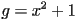. The first step is to cancel the first term of f by multiplying g by the appropriate term and substracting the result from f. When we do that we get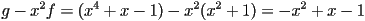. Doing this again we get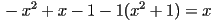. Now we won't be able to continue this process any further because if we multiply g by anything, even a constant, it will still have a higher degree than x. So the remainder when we divide f by g is x. In general, it is important to note that the degree of the remainder is always less than the degree of g. Now what would happen if wanted to divide by 2 polynomials,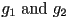? To be more precise, what we want to do is write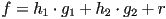where r has degree less than both. With some careful thought, one can show that you can find r by dividing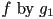and then dividing the remainder of that by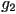. Also, one can prove that the result of this does not change if you switch the order in which you do the divisions.

One Variable Division
In this activity you get to test a case of the last statement. Let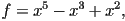andand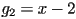and try dividing f by the other two polynomials in both orders. Do you get the same answer both times?
Psuedo-code is a term commonly used in computer science. This field is mainly focused on the theory (and practice) of how to get a computer to solve a problem you want it to solve. This is difficult because computers are actually much stupider than you might think. They do exactly what you tell them. For example, if I wrote a note to you and made a typo in it, like "Please clean teh car," you would understand what I meant. However, a computer would crash. Therefore, computer instructions, called code, must be written with extreme care. Psuedo-code is written so that a human can tell what it means precisely, but also so that it is much easier to read than code that is readable by computers.

Now you might wonder "is it possible to extend polynomial division to multiple variable polynomials in such a way that the remainder term is unique"? The answer is yes, but it is much more complicated than you might think. First let's look at the division algorithm in 1 variable in more detail. Here's a list of the steps we do to divide f by g, written in psuedo-code.

1. Let r = f.
2. if deg(r) < deg(g), return f
3. let n = deg(f) - deg(g), which is positive because of the previous line
4. replace r by the polynomial r - x^n * g
5. go back to line 2
Notice that this algorithm will return the remainder r of f divided by g, and this remainder will always have a degree that is smaller than g.

Now how could we extend this algorithm to polynomials with multiple variables? Well, in the second line it looks like we need a way to say whether one polynomial is less than another. The way to properly generalize this condition to multiple variables is subtle. What we actually want is a way to say whether 1 term (called a monomial) is less than another term. (Here we don't mean "less than" like "3 < 6", we mean a more general kind of "less than" called a "total ordering," which is described in the tangent.) Then to a polynomial f we assign the term in f which is the largest with respect to this ordering, which we call the leading term, or Lf. Then what we actually mean in the second line is "if Lg does not divide any term in f, return f." We'll get back to how to generalize the other lines after we discuss total orderings a bit.

A total ordering on a set is a function which inputs two variables and outputs either true or false. This function is supposed to behave in the same way that "<" does for actual numbers. For example, if a < b and b < c, then a < c, and for any a and b which are not the same, either a < b or b < a.

Now how do we assign a total order to monomials? With just one variable a monomial is just a constant times x^n, for some integer n, and it is easy to assign a total order, we just say x^n < x^m if n < m. However, if there are multiple variables, this becomes a little trickier. For example, which is "smaller," x or y? They both have the same degree, which is 1. Therefore, we must make some arbitrary choice, so for our examples we'll say x < y. Then we'll say x^a y^b < x^c y^d if either (a < c) or (a = c and b < d). So now we can compare monomials, which means we fully know how to do the second line.

Now we know that there is a monomial in f, which we'll call t, such that Lg divides t. Now let h = t / Lg, so that we can make f "smaller" by subtracting hg (because this will remove the term t). Now we are in a position to state the generalized algorithm, which you might already guess is significantly more complicated than our first algorithm.

1. Input: polynomials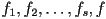2. Output: polynomials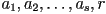3. .
4. set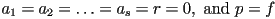5. WHILE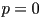DO
6. ___ set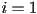and divisionoccured = false
7. ___ WHILE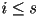AND divisionoccured = false DO
8. ___ ___ IFdivides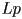THEN
9. ___ ___ ___ set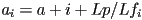10. ___ ___ ___ set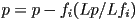11. ___ ___ ___ divisionoccured = true
12. ___ ___ ELSE
13. ___ ___ ___ set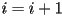14. ___ ___ If divisionoccured = false THEN
15. ___ ___ ___ set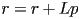16. ___ ___ ___ setThe output satisfies the following conditions: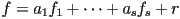, and the leading termsdo not divide any terms of r, for any i. We call r the remainder.

A bit of explanation is in order. The "WHILE C DO" command interacts with the indentation. It means that if the condition C is true, then you go to the next line, which is indented. Once you reach the end of the lines that are indented more than the WHILE, you go back to the beggining and check to see if C is true again. If C is false, then you go to the next line that is indented the same as the WHILE command is. The IF command is similar, except that once you reach the line that has the same indentation as it, you don't go back, you just keep on going forwards. This next activity will be more difficult than the previous one.

Multivariable Division
Now you get to try the second algorithm twice, with the following inputs:
1.2.Before you do the computations, notice that the only difference between the two inputs is that we've switched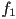and. Based on your experience in the previous activity, do you think you'll get the same remainder both times?

If you followed the algorithm correctly, you got different answers. This would cause significant problems in computations, which are a bit too theoretical to discuss here. However, Grobner bases are a way to fix this problem. Given a monomial ordering and input polynomials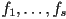, one can compute a new set of polynomialsso that the set where all the g polynomials are equal to 0 at once is equal to the set where all the f polynomials are equal to 0 at once (this is a very important condition in commutative algebra, but it's a bit too theoretical to explain here). Also, if you divide any polynomial h by the g's using the above algorithm, you will always get the same remainder.

In summary, Grobner bases are fairly technical, which is probably why they were only mentioned in passing on the show. They were invented in 1965, so they are a fairly recent invention as far as mathematics is concerned, and they have had a very important effect on both theoretical and practical mathematics. For theoretical math, it has made it much easier to compute explicit examples, which means it's easier to figure out new theoretical phenomena. It has also greatly improved the ability of computers to make computations with polynomials, which is important for various engineering applications, like tracking the movement of robot arms.